Win up to 100% scholarship on Aakash BYJU'S JEE/NEET courses with ABNAT Win up to 100% scholarship on Aakash BYJU'S JEE/NEET courses with ABNAT

# JEE Main 2021 July 20 Shift 1 Chemistry Question Paper

JEE Main 2021 Chemistry question paper for July 20 shift 1 exam is available here. In addition to the questions, students will also find solutions to each problem given in the paper. The solutions have been prepared by our subject experts and will help students to understand the correct answer.

### JEE Main 2021 July 20 Shift 1 Chemistry Question Paper

Going through the JEE Main 2021 July 20 shift 1 Chemistry questions and solutions will allow students to learn the problems quickly and easily. All in all, once they start solving the questions on their own they will be able to study productively for the upcoming entrance exam. Students can access the solution videos along with the JEE Main 2021 Physics question paper PDF for July 20 Shift 1 below.

July 20 Shift 1 – Chemistry Question Paper and Solutions

Question 1. The Vapour pressure of pure benzene is 70 torr and that of methylbenzene is 20 torr. If we have an equimolar mixture of the above two components, find the mole fraction of benzene in the vapour phase.

$$\begin{array}{l}P_{C_{6}H_{6}}^{0} = 70\: torr\end{array}$$
$$\begin{array}{l}P_{C_{6}H_{5}CH_{3}}^{0} = 20\: torr\end{array}$$
$$\begin{array}{l}X_{C_{6}H_{6}} = X_{C_{6}H_{5}CH_{3}}\end{array}$$
$$\begin{array}{l}Y_{C_{6}H_{6}} = \frac{P_{C_{6}H_{6}}^{0} \times X_{C_{6}H_{6}}}{(P_{C_{6}H_{6}}^{0}\times X_{C_{6}H_{6}}) + (P_{C_{6}H_{5}CH_{3}}^{0}\times X_{C_{6}H_{5}CH_{3}})}\end{array}$$
$$\begin{array}{l}Y_{C_{6}H_{6}} = \frac{70 \times X_{C_{6}H_{6}}}{(70\times X_{C_{6}H_{6}}) + (20\times X_{C_{6}H_{5}CH_{3}})}\end{array}$$
$$\begin{array}{l}Y_{C_{6}H_{6}} = \frac{7}{9}\end{array}$$

Question 2. The number of lone pairs present on the central atom of

$$\begin{array}{l}I_{3}^{-}\end{array}$$
is:

a. 9
b. 1
c. 6
d. 3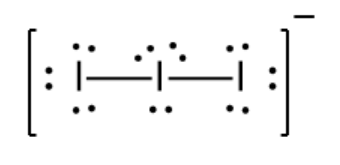$$\begin{array}{l}I_{3}^{-}\end{array}$$
has a linear structure. The number of lone pairs on the central atom is 3.

Question 3. The wave number of the spectral line in the emission spectrum of Hydrogen will be equal to 8/9 times the Rydberg’s constant if the electron jumps from:

a. n = 3 to n = 1
b. n = 9 to n = 1
c. n = 10 to n = 1
d. n = 2 to n = 1

Wave number

$$\begin{array}{l}(\bar{v})\end{array}$$
of spectral line in emission spectrum of hydrogen,

$$\begin{array}{l}(\bar{v}) = R_{H} \left ( \frac{1}{n_{1}^{2}}-\frac{1}{n_{2}^{2}} \right )\end{array}$$

Given:

$$\begin{array}{l}\bar{v} = \frac{8}{9}R_{H}\end{array}$$

Putting the value, we get:

$$\begin{array}{l}\frac{8}{9}R_{H} = R_{H} \left ( \frac{1}{n_{1}^{2}}-\frac{1}{n_{2}^{2}} \right )\end{array}$$
$$\begin{array}{l}\frac{8}{9} = \left ( \frac{1}{1^{2}} – \frac{1}{n_{2}^{2}} \right )\end{array}$$

N2 = 3

Question 4. 250 ml of 0.5 m NaOH is added to 500 mL of 1 M HCl. What is the final concentration of the remaining HCl?

a. 0.1 M
b. 0.5 M
c. 50 M
d. 5 M

Neutralization reaction:

NaOH + HCl → Nacl + H2O

Millimoles of NaOH = (250 × 0.5) mmol = 125 mmol

Millimoles of HCl = (500 × 1) mmol = 500 mmol

According to reaction:

1 mmol of HCl is neutralized by 1 mmol of NaOH.

HCl remaining = 500 – 25 mmol = 375 mmol

Final concentration of remaining

$$\begin{array}{l}HCl = \frac{HCl \: remaining}{Total\; Volume} = \frac{375 mmol}{(500+250)mL} = 0.5 M\end{array}$$

Question 5. Three complexes [CoCl(NH3)5]2+ (I), [Co(NH3)5H2O}3+ (II) and [Co(NH3)6}3+ (III)absorb light in the visible region. The correct order of the wavelengths of light absorbed by them is:

a. (III) > (I) > (II)
b. (I) > (II) > (III)
c. (II) > (I) > (III)
d. (III) > (II) > (I)

A complex having a strong field ligand has the tendency to absorb light of the highest energy. Among the three complexes, [Co(NH3)6}3+ will absorb radiation of the highest energy and that will have the least wavelength.

[Co(NH3)5H2O}3+ has a weaker field than the above compound and therefore absorbs radiation of lesser energy and more wavelength.

[CoCl(NH3)5]2+ has the weakest field and therefore will absorb light of least energy and highest wavelength.

Strength of ligand: NH3 > H2O > Cl

Question 6. A person requires 10000 kJ energy per day and ∆H combustion of glucose is 2700 kJ mol-1. How many grams of glucose are required by the body in a day?

a. 666.67 g
b. 6.67 g
c. 66.67 g
d. 6666.67 g

Molar mass of glucose (C6H12O6) = 180 g/mol

Moles of glucose required per day =

$$\begin{array}{l}\frac{1000\: kJ/day}{2700\: kJ/day} = \frac{100}{27}\: mol/day\end{array}$$

Grams of glucose required by the body in a day =

$$\begin{array}{l}\frac{100}{27}\times 180(\frac{g}{day})= 666.67\: g/day\end{array}$$

Question 7. Determine the product formed when 2,2-dimethyl-3-bromobutane reacts with ethanol at high temperatures.

a. 2,3-dimethylbut-2-ene
b. 3,3-dimethylbut-1-ene
c. 3-ethoxy-2,2-dimethylbutane
d. 2-ethoxy-2,3-dimethylbutane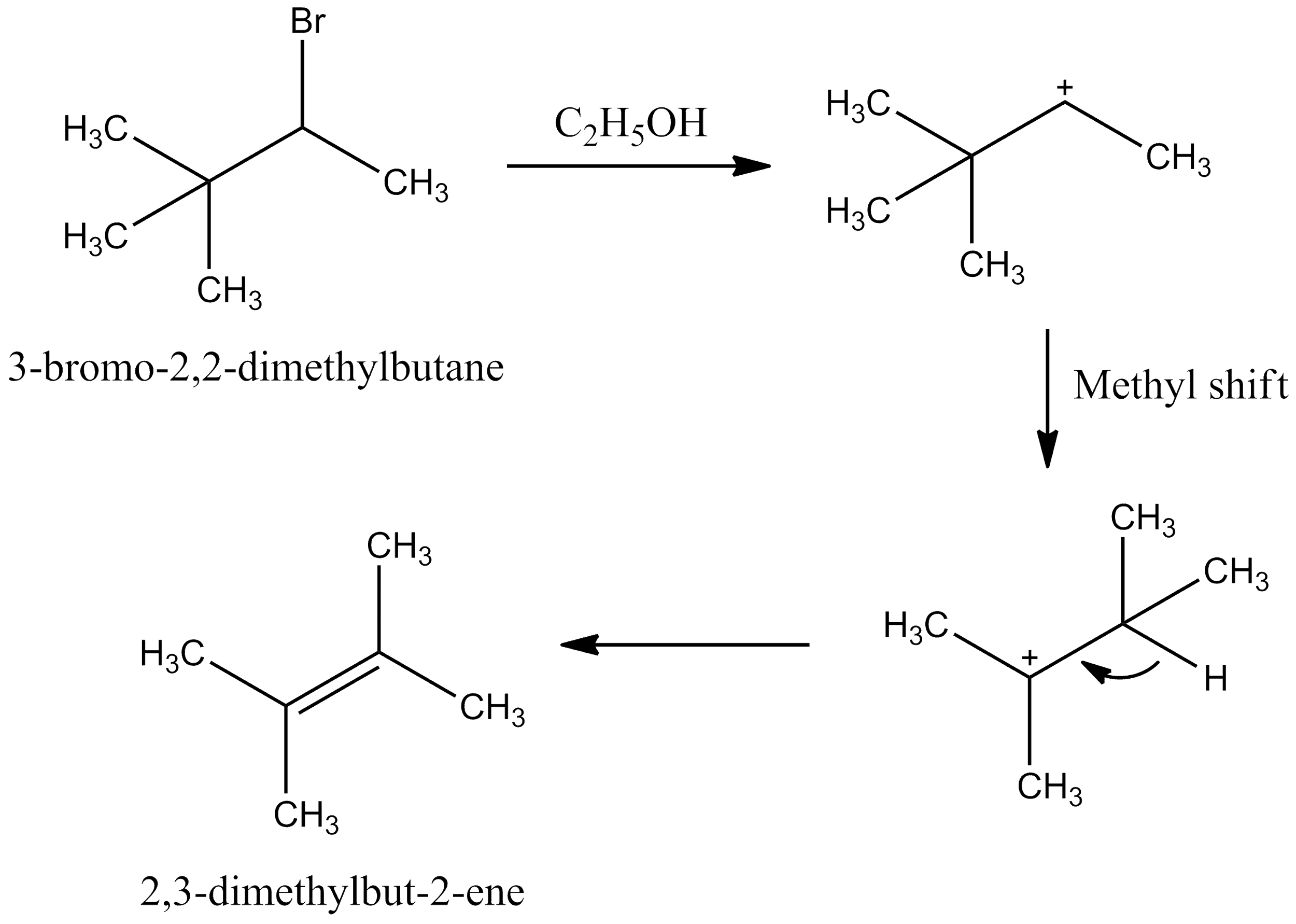Question 8. Ninhydrin test for proteins results in the structure: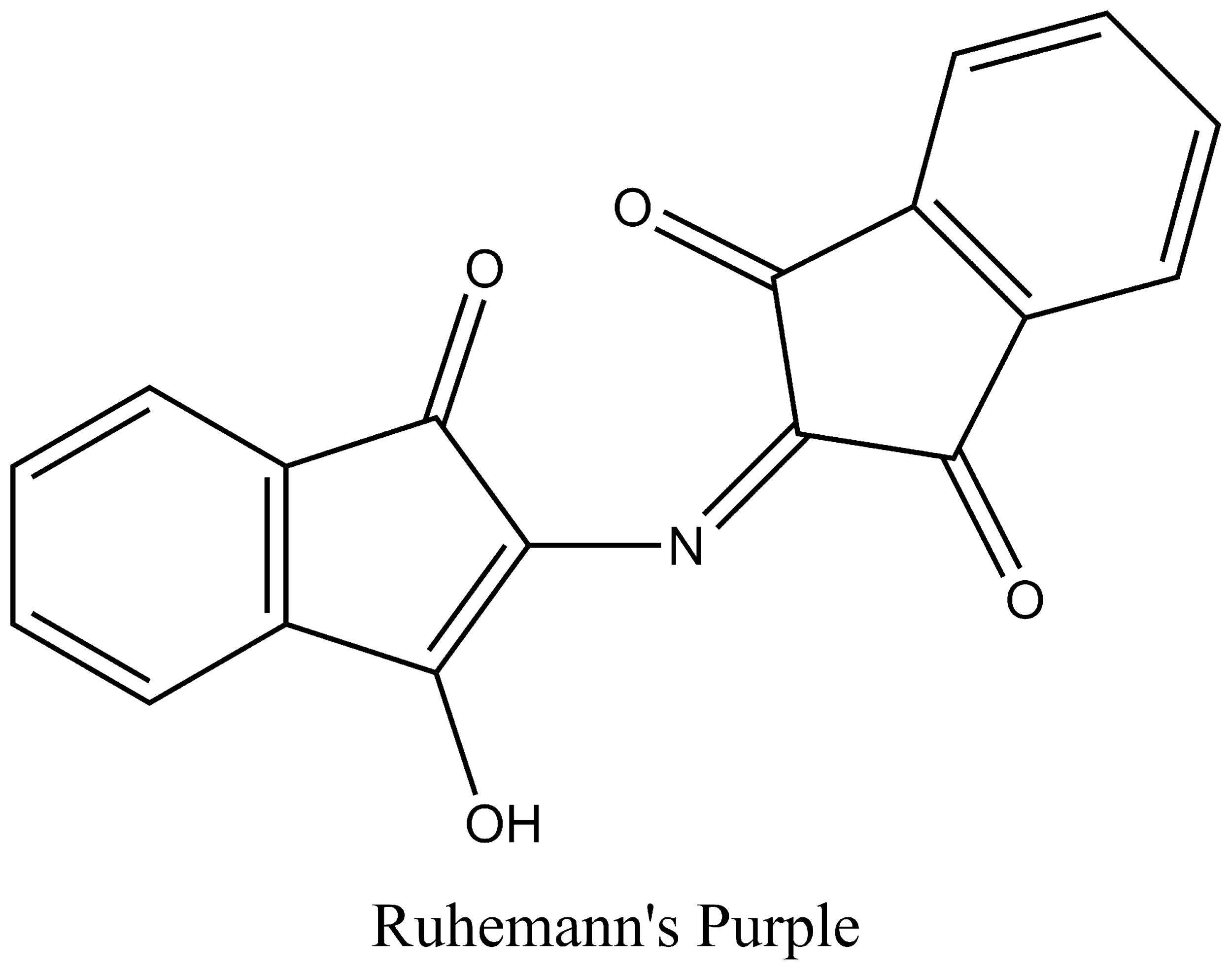Question 9. Which of the following is incorrect?

a. Amylose is branched
b. Glycogen is also called animal starch
c. Starch is made up of α-glucose

Amylose is a linear polysaccharide not branched.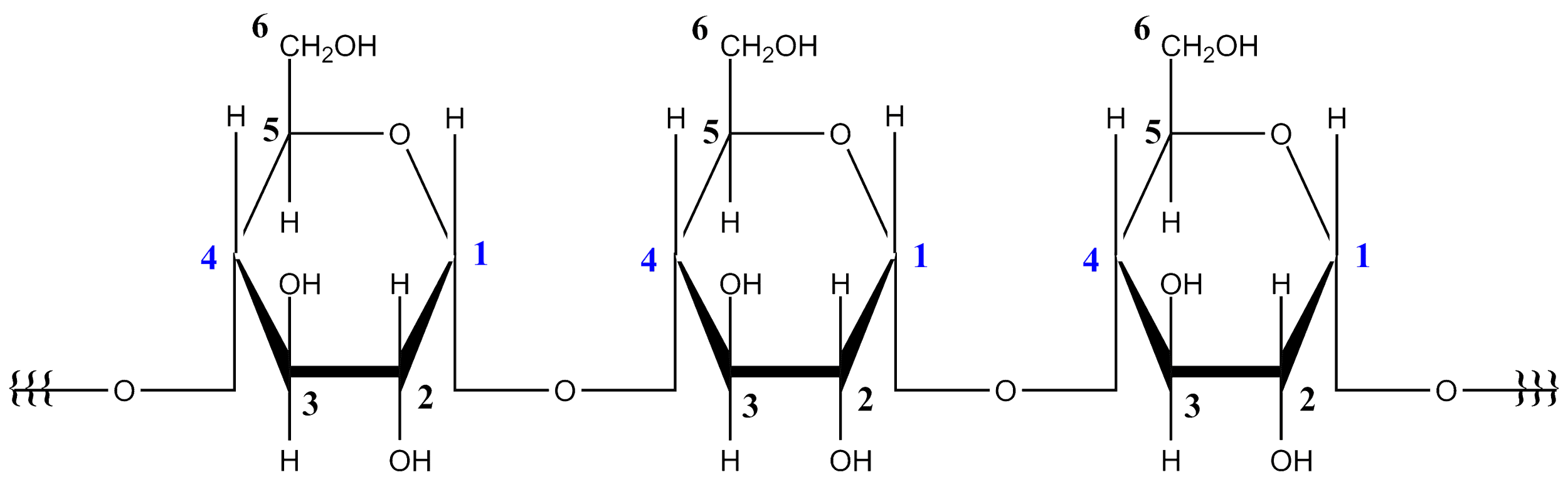Question 10. Which of the following will form superoxide?

a. K
b. Na
c. Mg
d. Ca

From group 1 elements, K, Rb, Cs can form Superoxide (MO2). Na cannot form superoxide, it can form peroxide. Mg and Ca do not form superoxides.

Question 11. Determine the number of nitrogen atoms in a semicarbazide.

a. 2
b. 3
c. 4
d. None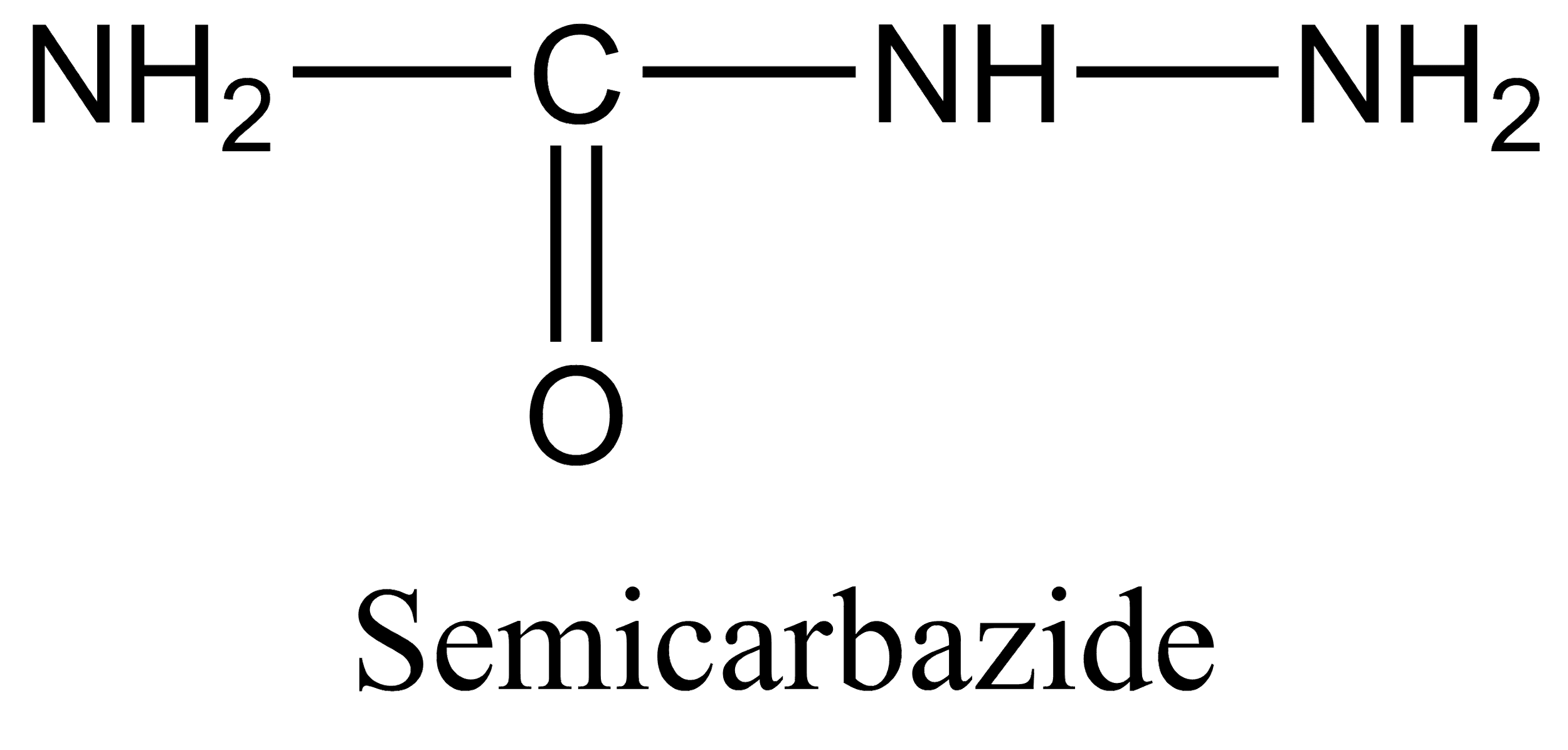Semicarbazide has 3 nitrogen atoms.

Question 12. How many equivalents of CH3MgBr are required to make 2-methylpropan-2-ol from ethyl ethanoate?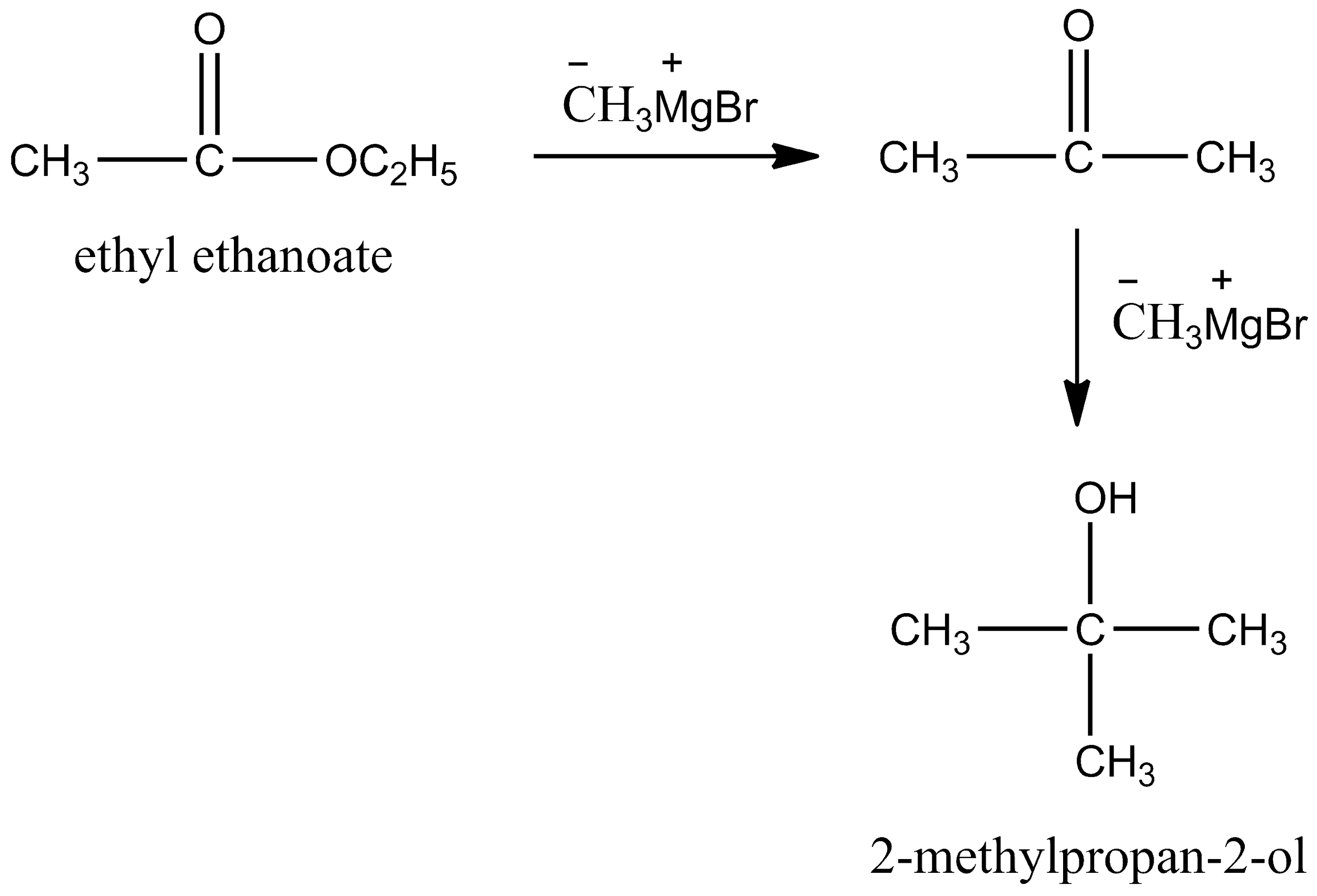Question 13. Which of the following does not disproportionate?

a. BrO
b.

$$\begin{array}{l}BrO_{2}^{-}\end{array}$$

c.
$$\begin{array}{l}BrO_{3}^{-}\end{array}$$

d.
$$\begin{array}{l}BrO_{4}^{-}\end{array}$$

For a species to undergo disproportionation, the oxidation state of the element undergoing disproportionation should lie between its maximum and minimum possible oxidation values.

In option (a), we have the oxidation state of Br = +1

In option (b), we have oxidation of Br = +3

In option (c), we have oxidation of Br = +5

The maximum and minimum possible oxidation state value for Br is +7 and -1 respectively. So, from this we can conclude that

$$\begin{array}{l}BrO_{4}^{-}\end{array}$$
will not undergo disproportionation.

Hence, option (d) is the correct answer.

Question 14. If P4O10 + HNO3 are mixed in a 1:4 ratio, then the nature of nitrogen oxide obtained will be:

a. Acidic
b. Basic
c. Amphoteric
d. Neutral

P4O10 + 4 HNO3 → 4HPO3 + 2N2O5

N2O5 is an acidic oxide.

Hence, option (a) is the correct answer.

Question 15. Which of the following is most economically refined by fractional distillation?

a. Zn
b. Ni
c. Cu
d. Fe

The main impurities in Zn are generally cadmium, lead and iron. There is very little difference in the boiling point of zinc and these impurities so zinc can be refined using fractional distillation.

For refining of copper, we generally use electrorefining, cupellation or poling as the refining techniques.

For Ni, vapour phase refining, i.e., the Mond process is used.

For Fe, we use an oxidative refining technique.

Hence, option (a) is the correct answer.

Question 16. Product A and B for the following reactions are as follows: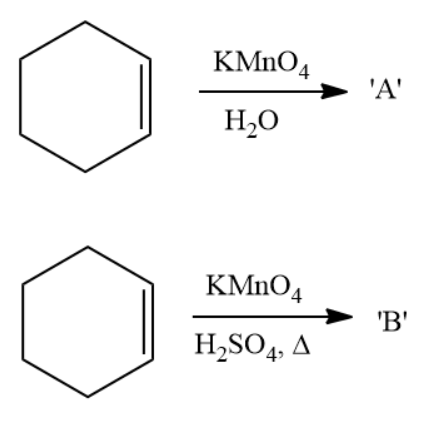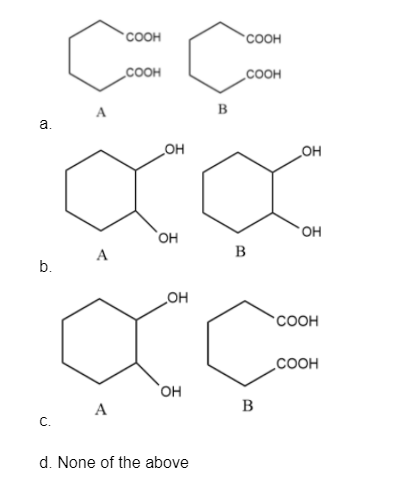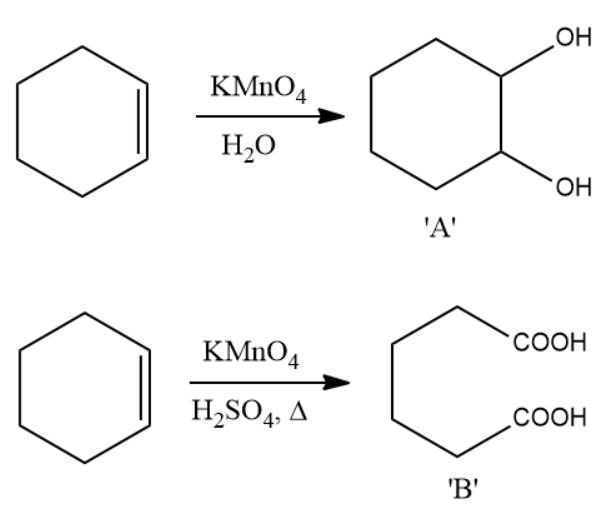Hence, option (c) is the correct answer.

Question 17. Determine the Azimuthal quantum number of the last electron of Ga+ ion.

a. 1
b. 0
c. 2
d. 3

31Ga – 1s2 2s22p63s23p63d104s24p1

31Ga+ – 1s2 2s22p63s23p63d104s2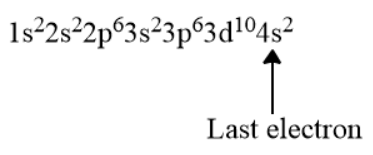Since the last electron is in the 4s subshell and for the ‘s’ subshell, the value of the azimuthal quantum number(l) is 0.

Hence, option (b) is the correct answer.

Question 18. In which of the following, resonance is not possible?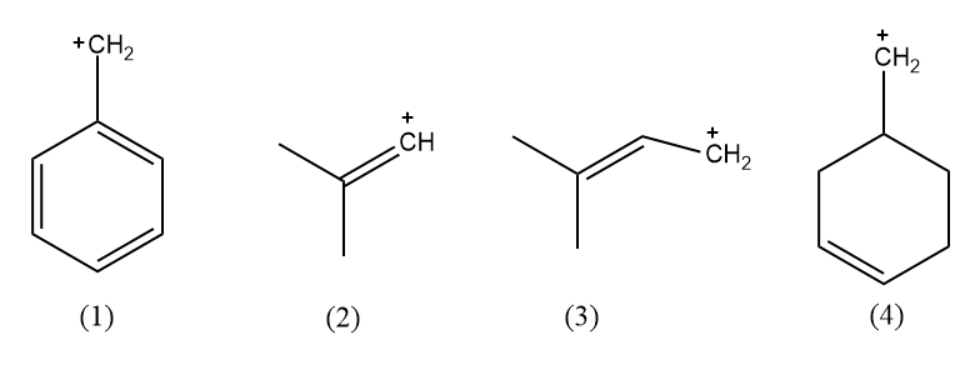a. 1, 2
b. 2, 4
c. 3, 4
d. 1, 4

Condition for resonance:

1. The Pi-bond is present at an alternate position to the atom bearing a vacant orbital.

eg.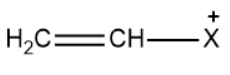2. The Pi-bond is present at the alternate position to the atom bearing a negative charge.

eg.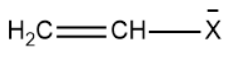3. A Pi-bond is present at the alternate position to the atom bearing a lone pair of electrons.

eg.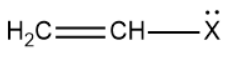4.Pi- bond present at the alternate position to the atom bearing lone electron.

eg.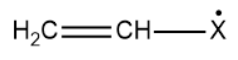5. A Pi-bond is present at the alternate position to another Pi-bond.

eg.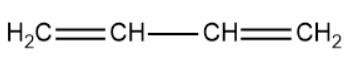Molecules (1) and (3) are obeying the above conditions.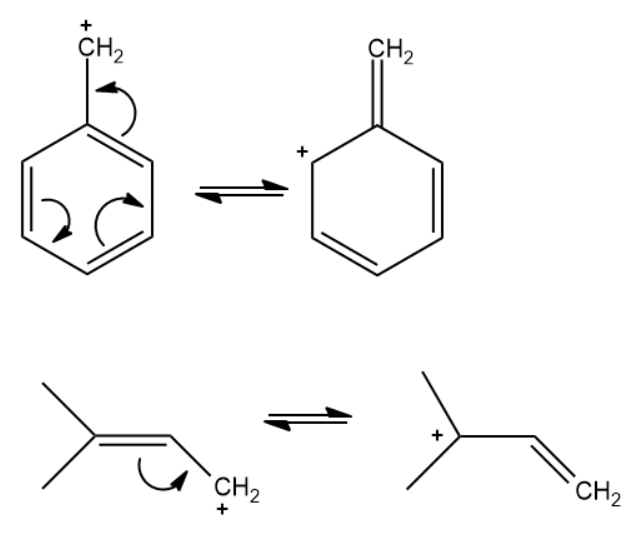Molecules (2) and (4) are not obeying any of these conditions mentioned above.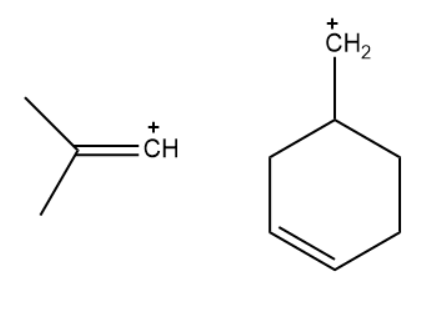Hence, option (b) is the correct answer.

Question 19. Which condition is required to show the Tyndall Effect by the colloidal solution?

a. The refractive indices of the dispersed phase and the dispersion medium differ greatly in magnitude
b. The refractive indices of the dispersed phase and the dispersion medium are exactly the same in magnitude
c. The refractive indices of the dispersed phase and the dispersion medium do not differ much in magnitude
d. None of these

For a colloidal solution to show the Tyndall effect:

The refractive indices of the dispersed phase and the dispersion medium should differ greatly in magnitude.

Hence, option (a) is the correct answer.

Question 20. Which of the following pairs do not have the same nature?

a. B(OH)3, H3PO3
b. Be(OH)2, Al(OH)3
c. B(OH)3, Al(OH)3
d. Mg(OH)2, NaOH

Option a: Both are acidic in nature.

B(OH)3 is orthoboric acid.

H3PO3 is phosphorous acid.

Option b: Both are amphoteric hydroxides

Option c: B(OH)3 is acidic and Al(OH)3 is amphoteric

Option d: Both are basic hydroxides

Hence, option (c) is the correct answer.

Question 21. Orlon is a polymer of

a. Acrylonitrile
b. Styrene
c. Vinyl chloride
d. None

Orlon is the commercial name for polyacrylonitrile. Therefore, orlon is the polymer of acrylonitrile.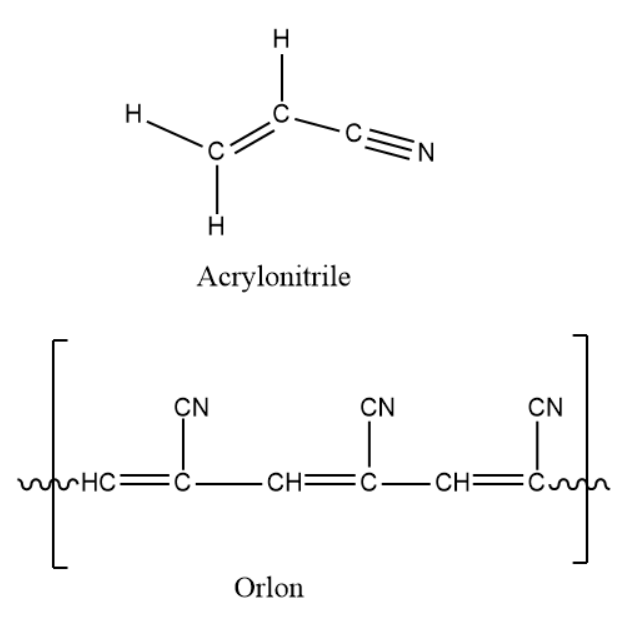Hence, option (a) is the correct answer.

Question 22. Are the statements true or false?

Statement-1: Dihedral angle of H2O2 in gas is 90°.

Statement-2: Dihedral angle of H2O2 in solid is 111°.

a. True, True
b. True, False
c. False, True
d. False, False

In a solid-state, the dihedral angle is smaller because of the presence of hydrogen bonding.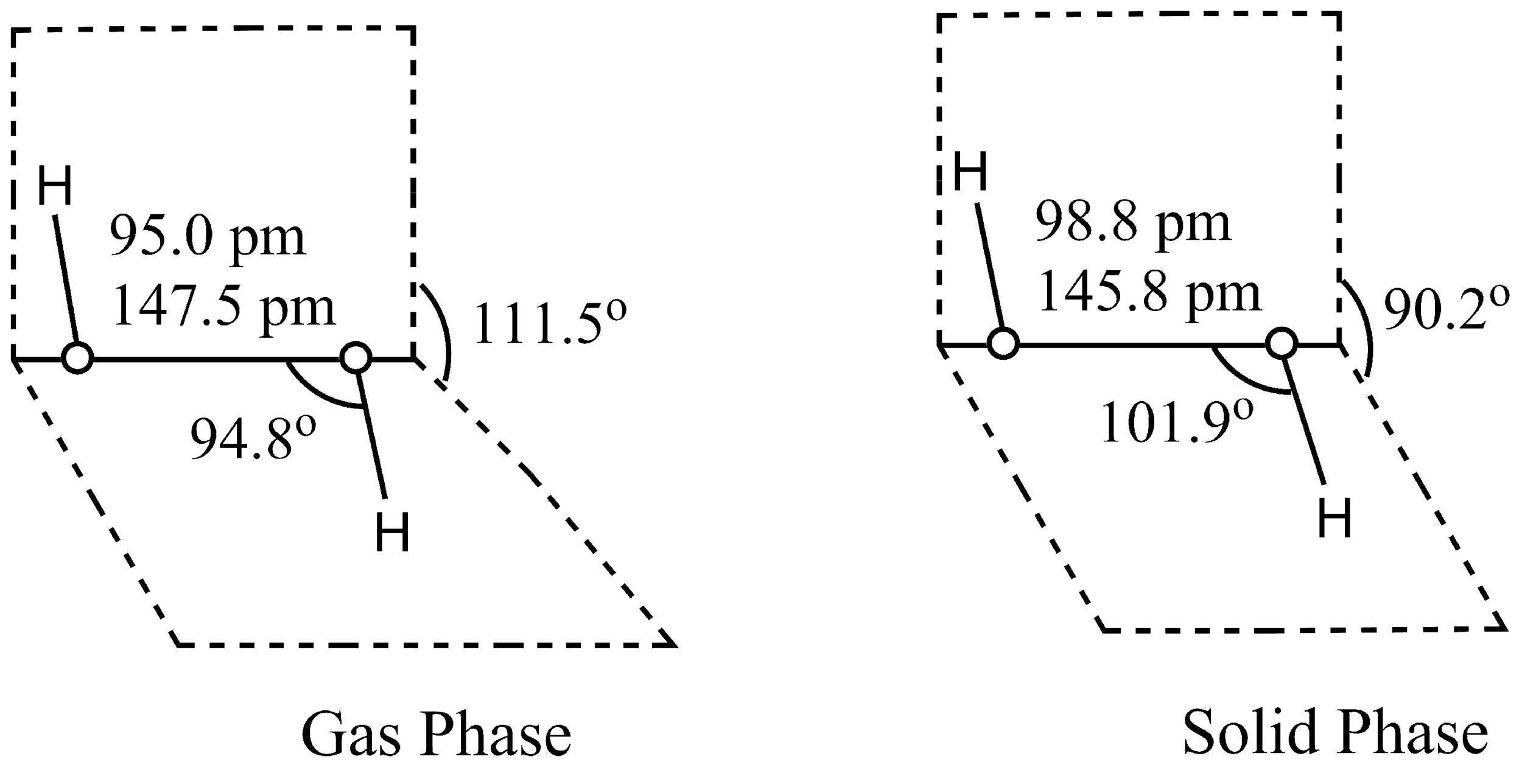Hence, option (d) is the correct answer.

Question 23. Green chemistry used in day-to-day life for:

a. Cleaning cloth with water<.p>
b. Using liquid H2O2 in dying clothes
c. Using tetrachloroethane in laundries
d. Using chlorine in bleaching paper

Green Chemistry is the branch of chemistry that deals with designing chemical products and processes that reduce or eliminate the use or generation of hazardous substances. Liquid H2O2 is a green reagent as it is used for dying clothes without generating any hazardous substances.

Hence, option (b) is the correct answer.

Question 24. The hybridization of xenon in XeOF4 is

a. sp3
b. sp3d
c. sp3d2
d. Sp3d3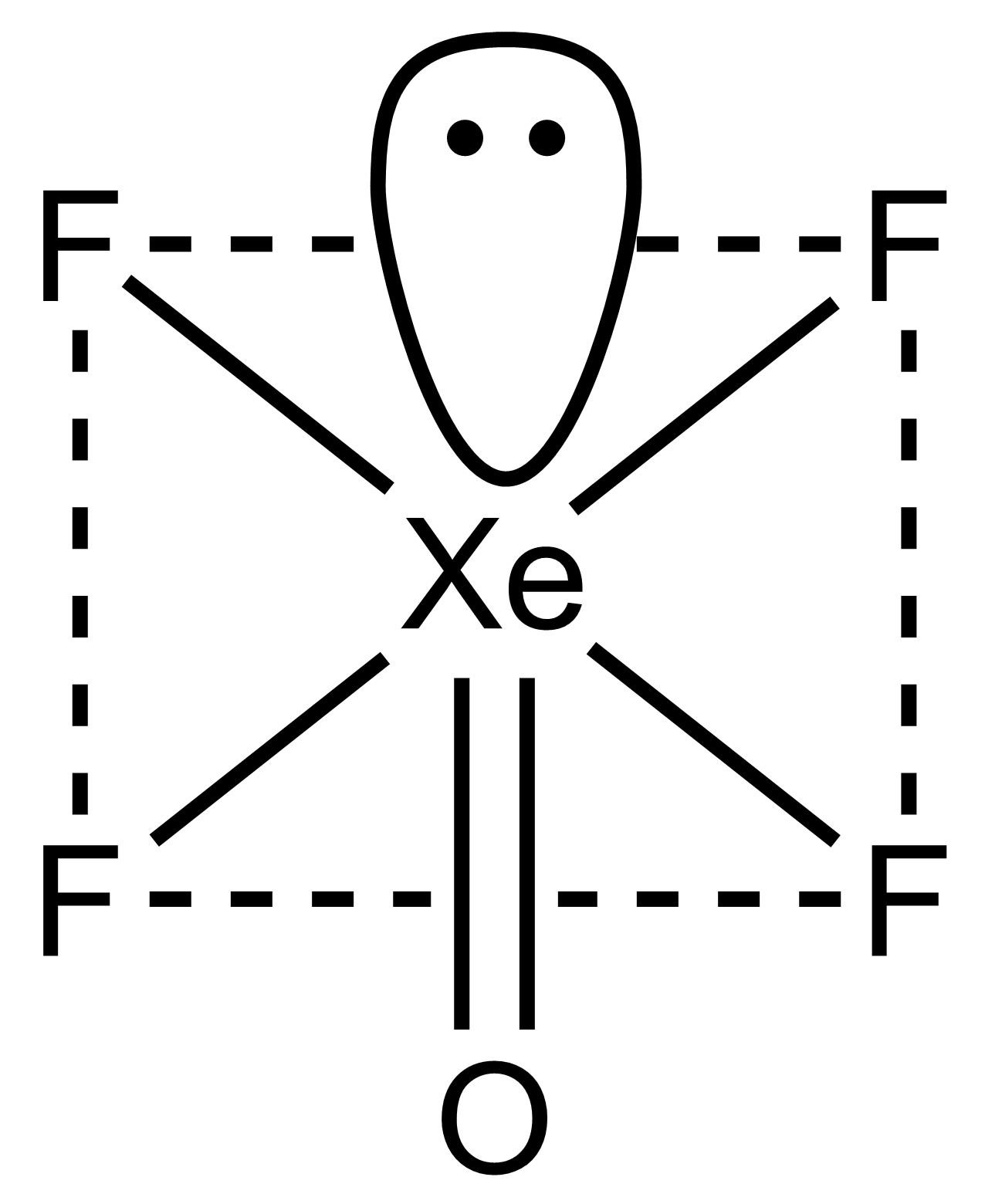Hybridization can be predicted with the help of a steric number.

Steric number = No. of sigma bond +No. of Lone pairs

Steric number = 5 + 1

Therefore,

The steric number is 6

So, the hybridization will be sp3d2

Hence, option (c) is the correct answer.

Question 25. Find the second excitation energy of Li2+.

a. 108.8 eV
b. 81.6 eV
c. 13.6 eV
d. 95.2 eV

Second excitation energy means the electron is jumping from the first shell to the third shell.

Therefore,

Second excitation energy, E = E3 – E1

E3 is energy in the third shell

E1 is energy in the first shell

Second excitation energy =

$$\begin{array}{l}\frac{-13.6 Z^{2}}{n_{3}^{2}} – \frac{-13.6 Z^{2}}{n_{1}^{2}}\end{array}$$
$$\begin{array}{l}E = -13.6 \times Z^{2}\left [ \frac{1}{n_{3}^{2}} – \frac{1}{n_{1}^{2}} \right ]\end{array}$$
$$\begin{array}{l}E = -13.6 \times 3^{2}\left [ \frac{1}{3^{2}} – \frac{1}{1^{1}} \right ]\end{array}$$
$$\begin{array}{l}E = -13.6 \times 3^{2}\left [ \frac{1}{9} – 1 \right ]\end{array}$$
$$\begin{array}{l}E = -13.6 \times 9\left [ \frac{-8}{9} \right ]\end{array}$$

E = 13.6 × 8

E = 108.8 eV

Hence, option (a) is the correct answer.